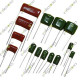Mylar Capacitors is special types of capacitors with some unique features compared with ceramic and electrolytic capacitors. They can withstand high voltages in a relatively small package and provides high resistance against moistureMylar capacitors are nonpolarized, meaning they can be connected in any direction.
Code J=+-5%
Code K=+-10%
Code M=+-20%
Code C=+-.25%

0.33nF 330p Mylar CapacitorCode = 331 ..
0.47nF 470pF 50V Mylar CapacitorCode = 471..
1nF 1000pF .001uF 102 100V Mylar CapacitorCode = 102 ..
1nF 1000pF .001uF 102 250V Mylar CapacitorCode = 102 ..
1nF 1000pF .001uF 102 400V  Mylar CapacitorCode = 102 ..
1nF 1000pF .001uF 102 630V Mylar CapacitorCode = 102 ..
1nF 1000pF .001uF 102 63V  Mylar CapacitorCode = 102 ..
1nF 1000pF .001uF 102 Mylar CapacitorCode = 102 ..
1.2nF 250V Mylar Capacitor..
1.2nF 2KV Mylar CapacitorCode = 122..
1.5nF 400V Mylar CapacitorCode = 152..
1.5nF Mylar CapacitorCode = 152 ..
1.8nF Mylar CapacitorCode = 182..
2.2nF 100V Mylar Capacitor..
2.2nF 50V Mylar Capacitor..
2.2nF 2200pF Mylar Capacitor Code = 222..
2.4nF 50V Mylar Capacitor..
2.7nF 50V Mylar Capacitor..
2.7nF 272 Mylar CapacitorCode = 272 ..
3nF 1KV Mylar Capacitor..
3nF 302 Mylar CapacitorCode = 302 ..
3.3nF 630V Mylar Capacitor..
3.3nF 3300pF 332 Mylar CapacitorCode = 332..
3.3nF Mylar Capacitor..
3.9nF 100V Mylar CapacitorCode = 392 Pin Distance: 0.5 cm Pin Length: 2.5 cm ..
3.9nF Mylar Capacitor Code = 392 ..
4.7nF 400V Mylar Capacitor Code = 472..
4.7nF 63V Mylar CapacitorCode = 472 ..
4.7nF Mylar CapacitorCode = 472 ..
4.7nF 50V Mylar CapacitorCode = 472 ..
Showing 1 to 30 of 125 (5 Pages)